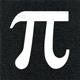# Math Is Fun Forum

Discussion about math, puzzles, games and fun.   Useful symbols: ÷ × ½ √ ∞ ≠ ≤ ≥ ≈ ⇒ ± ∈ Δ θ ∴ ∑ ∫  π  -¹ ² ³ °

You are not logged in.

## #1 2015-10-23 20:22:58

zetafunc
ModeratorRegistered: 2014-05-21
Posts: 2,361
Website

### Abel Summation (Summation by Parts)

ABEL SUMMATION: The Continuous Version of the Summation by Parts Formula

Let
be an arithmetic function. Denote the partial sums of a by:

Let
, and suppose that
is a continuously differentiable function on
. Then:

One of the many applications of this theorem is using it to show that:

by writing

where
denotes the greatest integer part of x, and
is the Euler-Mascheroni constant.

Last edited by zetafunc (2015-10-27 07:22:34)

Offline